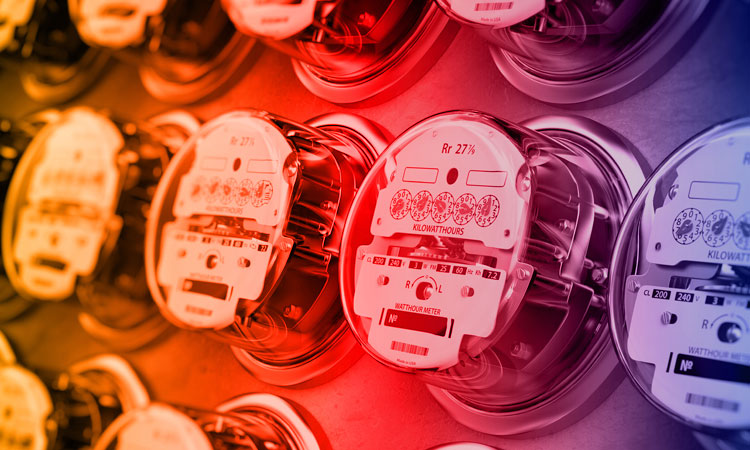# Kilowatts and Kilowatt-Hours

## Kilowatts and Kilowatt-HoursSimply stated, kW is kilowatts or power; kWh is kilowatt-hours or energy used over a period of time. For simplicity, we will refer to kW as power (also referred to as capacity, demand or load) and kWh as energy (also referred to as consumption or usage). The kW capacity or demand is the rate at which energy is consumed.

The following example may be helpful. A single 60-watt light bulb operating for 10 hours would use 600 watt-hours or 0.6 kWh of energy. This is exactly the same amount of energy that is used if six 100-watt light bulbs were operated for one hour. However, the electrical capacity or demand required to supply six 100-watt light bulbs would be 10 times greater than that required to operate the single 60-watt light bulb.

• One 60-watt light bulb for 10 hours: Demand = 60W/1000 = 0.06kW, Energy = 60W/1000 * 10 hours = 0.6kWh
• Six 100-watt light bulbs for one hour: Demand = 6 * 100W / 1000 = 0.6kW; Energy = 6 * 100W / 1000 = 0.6kWh

The same principle applies to how residential members use their household appliances. If someone bakes cookies during the same time period that they dry clothes, the electrical demand will be the sum of the individual demands of each appliance. The same as with the six light bulbs, the kW demand in this case will be much higher than if that same person bakes cookies, turns off their oven and then starts the dryer.

Here is an example: In the first case, where both appliances are in use at the same time, the total kW demand is the sum of demands of both appliances. That is 3.8kW plus 5.5 kW, or 9.3 kW. Since both appliances are on for an entire hour in our example, the total energy use is 9.3 kW x 1 hour or 9.3 kWh. In the second case, where the stove and dryer are not in use at the same time, the kW demand will NOT be the sum of the demands, but instead reflect the highest demand of the two appliances, i.e., the 5.5 kW that is required for the dryer. However, the total energy consumed is 9.3 kWh in both scenarios.

• 3800-watt oven and 5500-watt clothes dryer for one hour: Demand = 3800 + 5500 / 1000 = 9.3kW, Energy = 3800 + 5500 / 1000 * 1 hour = 9.3kWh
• 3800-watt oven for one hour: Demand = 3800 / 1000 = 3.8kW, Energy = 3800 / 1000 * 1 hour = 3.8kWh
• 5500-watt clothes dryer for one hour: Demand = 5500 / 1000 = 5.5kW, Energy = 5500 / 1000 * 1 hour = 5.5kWh